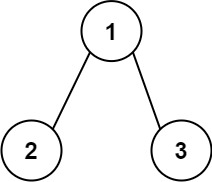## C# || How To Get Total Sum Root To Leaf Numbers In Binary Tree Using C#The following is a module with functions which demonstrates how to get the total sum root to leaf numbers in a binary tree using C#.

1. Sum Numbers – Problem Statement

You are given the root of a binary tree containing digits from 0 to 9 only.

Each root-to-leaf path in the tree represents a number.

• For example, the root-to-leaf path 1 -> 2 -> 3 represents the number 123.

Return the total sum of all root-to-leaf numbers. Test cases are generated so that the answer will fit in a 32-bit integer.

A leaf node is a node with no children.

Example 1:``` Input: root = [1,2,3] Output: 25 Explanation: The root-to-leaf path 1->2 represents the number 12. The root-to-leaf path 1->3 represents the number 13. Therefore, sum = 12 + 13 = 25. ```

Example 2:``` Input: root = [4,9,0,5,1] Output: 1026 Explanation: The root-to-leaf path 4->9->5 represents the number 495. The root-to-leaf path 4->9->1 represents the number 491. The root-to-leaf path 4->0 represents the number 40. Therefore, sum = 495 + 491 + 40 = 1026. ```

2. Sum Numbers – Solution

The following are two solutions which demonstrates how to get the total sum root to leaf numbers in a binary tree.

Both solutions use Depth First Search to explore items at each level.

Recursive

``` 2. Sum Numbers - Recursive - Solution C# // ============================================================================ // Author: Kenneth Perkins // Date: Nov 3, 2021 // Taken From: http://programmingnotes.org/ // File: Solution.cs // Description: Demonstrates how to get sum root to leaf numbers // ============================================================================ /** * Definition for a binary tree node. * public class TreeNode { * public int val; * public TreeNode left; * public TreeNode right; * public TreeNode(int val=0, TreeNode left=null, TreeNode right=null) { * this.val = val; * this.left = left; * this.right = right; * } * } */ public class Solution { public int SumNumbers(TreeNode root) { return Traverse(root, 0); } private int Traverse(TreeNode node, int currentSum) { if (node == null) { return 0; } // Calculate current sum currentSum = (currentSum * 10) + node.val; // We have reached a leaf node if (node.left == null && node.right == null) { return currentSum; } // Keep traversing left and right calculating the sum return Traverse(node.left, currentSum) + Traverse(node.right, currentSum); } }// http://programmingnotes.org/ 123456789101112131415161718192021222324252627282930313233343536373839404142 // ============================================================================//    Author: Kenneth Perkins//    Date:   Nov 3, 2021//    Taken From: http://programmingnotes.org///    File:  Solution.cs//    Description: Demonstrates how to get sum root to leaf numbers// ============================================================================/** * Definition for a binary tree node. * public class TreeNode { *     public int val; *     public TreeNode left; *     public TreeNode right; *     public TreeNode(int val=0, TreeNode left=null, TreeNode right=null) { *         this.val = val; *         this.left = left; *         this.right = right; *     } * } */public class Solution {    public int SumNumbers(TreeNode root) {        return Traverse(root, 0);    }     private int Traverse(TreeNode node, int currentSum) {        if (node == null) {            return 0;        }         // Calculate current sum        currentSum = (currentSum * 10) + node.val;         // We have reached a leaf node        if (node.left == null && node.right == null) {            return currentSum;        }         // Keep traversing left and right calculating the sum        return Traverse(node.left, currentSum) + Traverse(node.right, currentSum);    }}// http://programmingnotes.org/ ```

Iterative

``` 2. Sum Numbers - Iterative - Solution C# // ============================================================================ // Author: Kenneth Perkins // Date: Nov 3, 2021 // Taken From: http://programmingnotes.org/ // File: Solution.cs // Description: Demonstrates how to get sum root to leaf numbers // ============================================================================ /** * Definition for a binary tree node. * public class TreeNode { * public int val; * public TreeNode left; * public TreeNode right; * public TreeNode(int val=0, TreeNode left=null, TreeNode right=null) { * this.val = val; * this.left = left; * this.right = right; * } * } */ public class Solution { public int SumNumbers(TreeNode root) { if (root == null) { return 0; } // Declare stack var stack = new Stack<KeyValuePair<TreeNode, int>>(); var result = 0; // Add root to stack stack.Push(new KeyValuePair<TreeNode, int>(root, 0)); // Loop through items on the stack while (stack.Count > 0) { // Get current node and running sum var current = stack.Pop(); var node = current.Key; var currentSum = current.Value; // Calculate current sum currentSum = (currentSum * 10) + node.val; // We have reached a leaf node if (node.left == null && node.right == null) { result += currentSum; } else { // Keep traversing left and right calculating the sum if (node.left != null) { stack.Push(new KeyValuePair<TreeNode, int>(node.left, currentSum)); } if (node.right != null) { stack.Push(new KeyValuePair<TreeNode, int>(node.right, currentSum)); } } } return result; } }// http://programmingnotes.org/ 12345678910111213141516171819202122232425262728293031323334353637383940414243444546474849505152535455565758 // ============================================================================//    Author: Kenneth Perkins//    Date:   Nov 3, 2021//    Taken From: http://programmingnotes.org///    File:  Solution.cs//    Description: Demonstrates how to get sum root to leaf numbers// ============================================================================/** * Definition for a binary tree node. * public class TreeNode { *     public int val; *     public TreeNode left; *     public TreeNode right; *     public TreeNode(int val=0, TreeNode left=null, TreeNode right=null) { *         this.val = val; *         this.left = left; *         this.right = right; *     } * } */public class Solution {    public int SumNumbers(TreeNode root) {        if (root == null) {            return 0;        }        // Declare stack        var stack = new Stack<KeyValuePair<TreeNode, int>>();        var result = 0;         // Add root to stack        stack.Push(new KeyValuePair<TreeNode, int>(root, 0));         // Loop through items on the stack        while (stack.Count > 0) {            // Get current node and running sum            var current = stack.Pop();            var node = current.Key;            var currentSum = current.Value;             // Calculate current sum            currentSum = (currentSum * 10) + node.val;             // We have reached a leaf node            if (node.left == null && node.right == null) {                result += currentSum;            } else {                // Keep traversing left and right calculating the sum                if (node.left != null) {                    stack.Push(new KeyValuePair<TreeNode, int>(node.left, currentSum));                }                if (node.right != null) {                    stack.Push(new KeyValuePair<TreeNode, int>(node.right, currentSum));                }            }        }        return result;    }}// http://programmingnotes.org/ ```

QUICK NOTES:
The highlighted lines are sections of interest to look out for.

The code is heavily commented, so no further insight is necessary. If you have any questions, feel free to leave a comment below.

Once compiled, you should get this as your output for the example cases:

``` 25 1026 ```